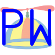PropWare  3.0.0.229 C++ objects and CMake build system for Parallax Propeller
StringBuilder_Demo.cpp

Compare the static and dynamic string build classes along with an unbuffered formatted print to the serial terminal.

cmake_minimum_required(VERSION 3.12)
find_package(PropWare REQUIRED)
project(StringBuilder_Demo)
create_simple_executable(\${PROJECT_NAME} StringBuilder_Demo.cpp)
static unsigned int next_fibonacci (const bool clear = false);
static unsigned int run_buffered (const int iterations);
static unsigned int run_static_buffered (const int iterations);
static unsigned int run_unbuffered (const int iterations);
int main () {
unsigned int dynamicBuffer, staticBuffer, noBuffer;
pwOut << "PropWare::StringBuilder Demo\n";
pwOut << "============================\n";
// Get iterations
int iterations;
pwIn.input_prompt("How many iterations of the fibonacci sequency would you like to see?\n>>> ",
"Please enter a non-negative whole-number\n", &iterations, NON_NEGATIVE_COMP);
// Run with StringBuilder
dynamicBuffer = run_buffered(iterations);
pwOut << "Total runtime was " << dynamicBuffer << "us using the PropWare::StringBuilder class\n";
// Reset the fibonacci function
pwOut << "\nLet's try this again, but static initialization (no use of malloc).\n";
next_fibonacci(true);
// Run with StaticStringBuilder
staticBuffer = run_static_buffered(iterations);
pwOut << "Total runtime was " << staticBuffer << "us using the PropWare::StaticStringBuilder class\n";
// Reset the fibonacci function
pwOut << "\nFinally, let's try this without any buffering.\n";
next_fibonacci(true);
// Run unbuffered
noBuffer = run_unbuffered(iterations);
pwOut << "Total runtime was " << noBuffer << "us without buffering\n";
// Exit
pwOut.println("Runtime results:");
pwOut << "\tDynamic buffer: " << dynamicBuffer << " us\n";
pwOut << "\tStatic buffer: " << staticBuffer << " us\n";
pwOut << "\tUnbuffered: " << noBuffer << " us\n";
return 0;
}
unsigned int run_buffered (const int iterations) {
const unsigned int timerStart = CNT;
StringBuilder string;
const Printer stringStream(string);
for (int i = 0; i < iterations; ++i) {
stringStream << next_fibonacci();
if (i != iterations - 1)
stringStream << ", ";
}
pwOut << string.to_string() << '\n';
return Utility::measure_time_interval(timerStart);
}
unsigned int run_static_buffered (const int iterations) {
const unsigned int timerStart = CNT;
char buffer;
StaticStringBuilder string(buffer);
const Printer stringStream(string);
for (int i = 0; i < iterations; ++i) {
stringStream << next_fibonacci();
if (i != iterations - 1)
stringStream << ", ";
}
pwOut << string.to_string() << '\n';
return Utility::measure_time_interval(timerStart);
}
unsigned int run_unbuffered (const int iterations) {
const unsigned int timerStart = CNT;
for (int i = 0; i < iterations; ++i) {
pwOut << next_fibonacci();
if (i != iterations - 1)
pwOut << ", ";
}
pwOut << '\n';
return Utility::measure_time_interval(timerStart);
}
unsigned int next_fibonacci (const bool clear) {
static unsigned int next = 1;
static unsigned previous = 1;
if (clear) {
next = 1;
previous = 1;
} else {
const unsigned int retVal = next;
next += previous;
previous = retVal;
return retVal;
}
return 0;
}
PropWare::StaticStringBuilder
Build a statically-sized string in RAM using the PropWare::Printer interface.
Definition: staticstringbuilder.h:36
printer.h
clear
int clear(void)
Clear the display.
Definition: oled_clear.c:7
stringbuilder.h
NON_NEGATIVE_COMP
const PropWare::NonNegativeIntegerComparator NON_NEGATIVE_COMP
Global instance for shared use by PropWare applications.
Definition: comparator.cpp:28
PropWare::Utility
Definition: utility.h:35
staticstringbuilder.h
PropWare::Scanner::input_prompt
void input_prompt(const char prompt[], const char failureResponse[], char userInput[], const size_t bufferLength, const Comparator< char > &comparator)
Prompt the user for input and store the value only if it is sanitized.
Definition: scanner.h:311
main
int main(void)
Definition: GraphicsTest.c:20
PropWare.h
string
void string(char *str)
Display a character string on the oLED display.
Definition: oled_string.c:7
scanner.h
pwOut
PropWare::Printer pwOut
Most common use of printing in PropWare applications (not thread safe; see PropWare::pwSyncOut for mu...
CNT
#define CNT
The system clock count.
Definition: propeller1.h:151
PropWare::StringBuilder
Build a dynamically-sized string in RAM using the PropWare::Printer interface.
Definition: stringbuilder.h:37
PropWare::Printer
Container class that has formatting methods for human-readable output. This class can be constructed ...
Definition: printer.h:76
PropWare
Generic definitions and functions for the Parallax Propeller.
Definition: runnable.h:33
PropWare::Printer::println
void println(const char string[]) const
Print a null-terminated string followed by a newline (' ')
Definition: printer.h:599